# Area of squares formed by joining mid points repeatedly in C Program?

CServer Side ProgrammingProgramming

Suppose we have one square whose side is ‘a’. We will make more squares by attaching the mid-points of the squares repeatedly. The number of repetition is n times. We have to find the area of nth square.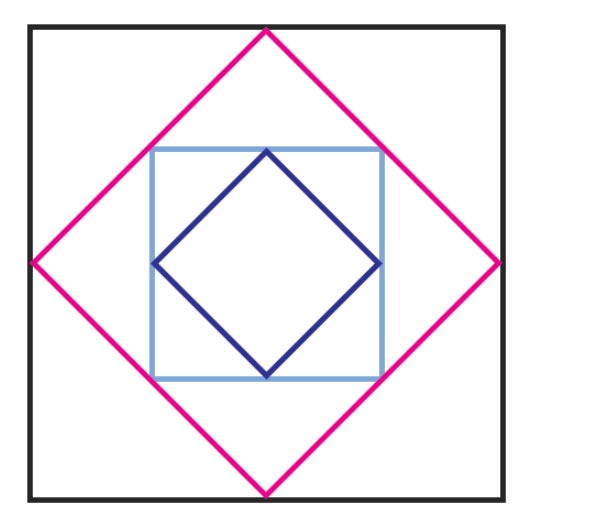As the side of the outer square is ‘a’, then area is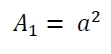Now using Pythagorean theorem, we can get the area of the second rectangle is −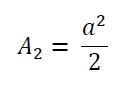Similarly, area of 3rd square is −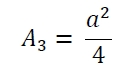Using this we can understand that the area of nth square is −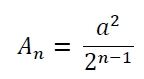## Example

#include <iostream>
#include <cmath>
using namespace std;
float area(float a, float n) {
if (a < 0 ) //if the value is negative it is invalid
return -1;
float area = (a*a) / pow(2, n-1);
return area;
}
int main() {
float a = 20.0, n = 10.0;
cout << "Area : " << area(a, n);
}

## Output

Area : 0.78125